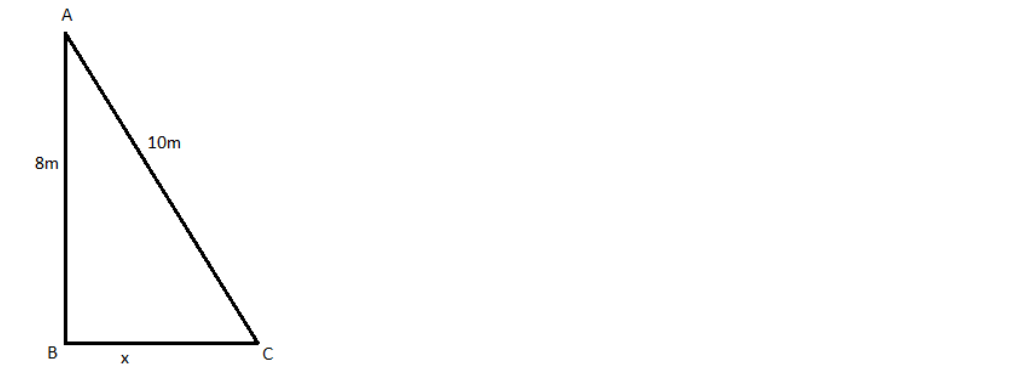QuestionAnswers

# A ladder ${\text{10m}}$long reaches a window ${\text{8m}}$ above the ground. Find the distance of the foot of the ladder from the base of the wall.Verified
131.4k+ views
Hint: We can draw a figure of the given situation as a right-angled triangle. Then we can find the unknown side using the Pythagoras theorem.

We can draw the figure with the given details.Let A be the window of the house, B be the base of the house and C be the foot of the ladder. This forms a right-angled triangle.
It is given that length of ladder is ${\text{10m}}$. So, ${\text{AC = 10m}}$.
The window is ${\text{8m}}$high. So ${\text{AB = 8m}}$.
We need to find the distance of the foot of the ladder from the base of the wall.
Consider the right triangle ABC. According to Pythagoras theorem, for a right-angled triangle,
${\text{A}}{{\text{B}}^{\text{2}}}{\text{ + B}}{{\text{C}}^{\text{2}}}{\text{ = A}}{{\text{C}}^{\text{2}}}$
Substituting the values of AB and AC in the equations, we get,
${{\text{8}}^{\text{2}}}{\text{ + B}}{{\text{C}}^{\text{2}}}{\text{ = 1}}{{\text{0}}^{\text{2}}}$
Subtracting both sides with ${{\text{8}}^{\text{2}}}$, we get,
${\text{B}}{{\text{C}}^{\text{2}}}{\text{ = 1}}{{\text{0}}^{\text{2}}}{\text{ - }}{{\text{8}}^{\text{2}}}$
Taking the squares and simplifying, we get,
${\text{B}}{{\text{C}}^{\text{2}}}{\text{ = 100 - 64 = 36}}$
Taking the square root, we get,
${\text{BC = }}\sqrt {{\text{36}}} {\text{ =6}}$
As we are taking the distance, we only take the positive value.
$\Rightarrow {\text{BC = 6m}}$
Therefore, the distance of the foot of the ladder from the base of the wall is ${\text{6m}}$.

Note: This problem is solved using Pythagoras theorem. According to the Pythagoras theorem, for any right-angled triangle, the sum of the squares of its non-hypotenuse sides is equal to the square of the hypotenuse. We cannot use trigonometry here as we are not given the details of any of the angles other than the right angle.